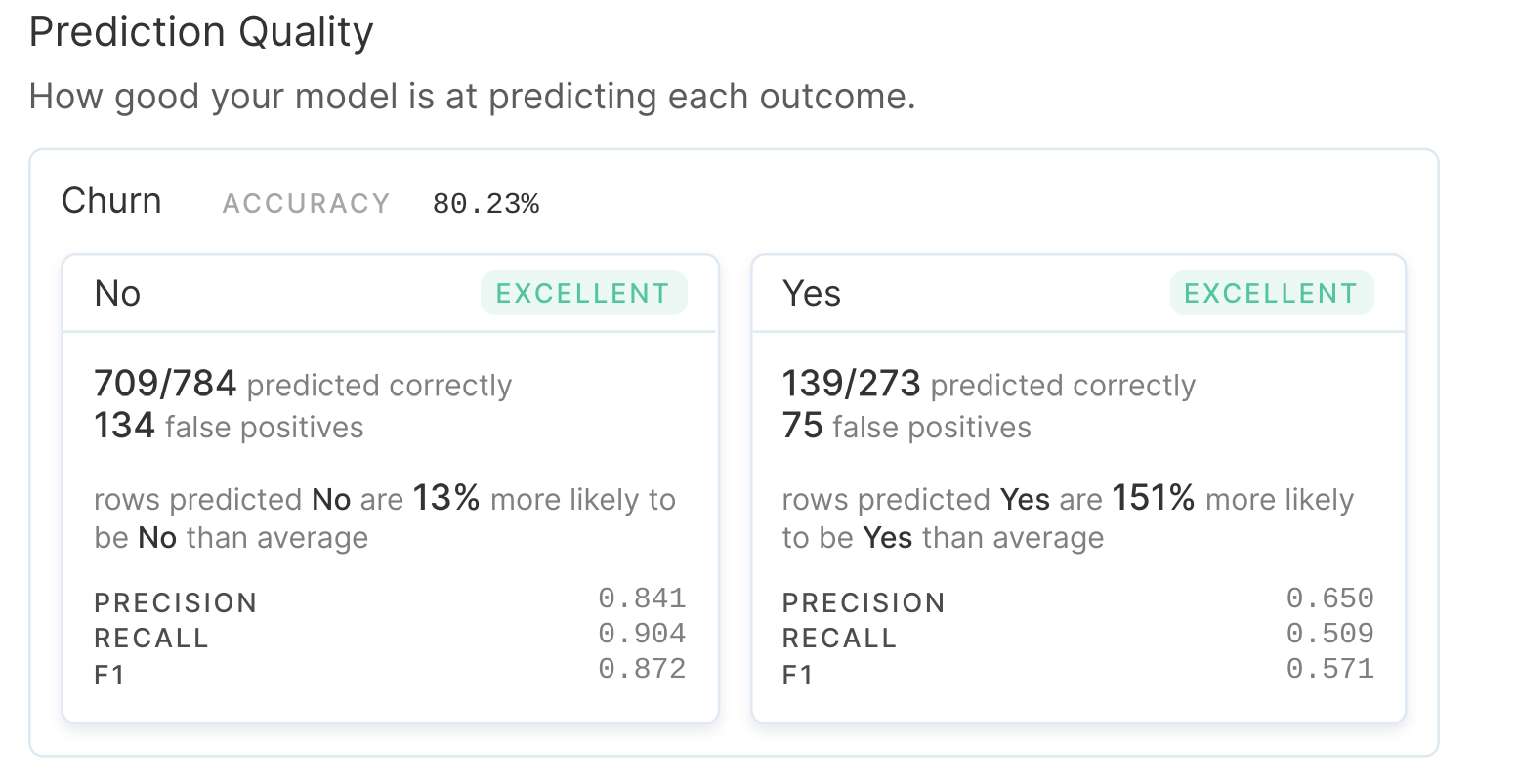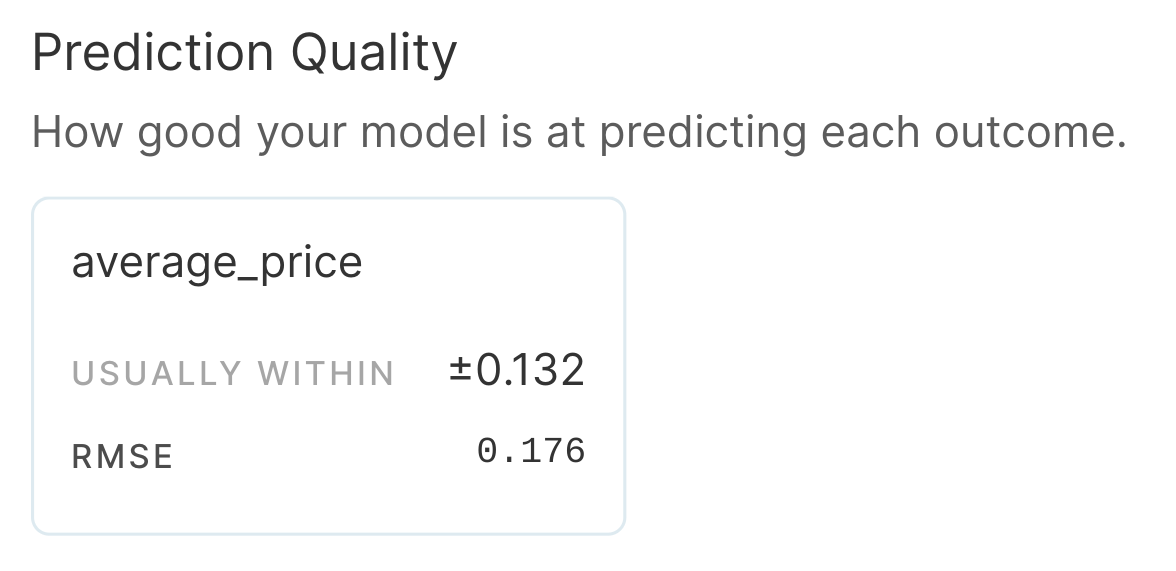# Model Performance

After building a model, you can view the “Prediction Quality” section of a model report to understand the model’s performance.
The prediction quality will have varying metrics, depending on if you built a classification or forecasting model.
Classification prediction quality includes percentage accuracy, precision, recall, and F1 score, as well as the number of values predicted correctly and incorrectly for each class. An example of “Prediction Quality” for a classification model is given below.Here are the definitions for these fields:
• Accuracy: Accuracy is a metric that measures how often a prediction is correct. It is calculated by dividing the number of predictions that were correct by the total number of predictions made.
• Precision: Precision is the fraction of true positives in your model’s predicted positives. This is useful to consider when the cost of a false positive is high, such as in email spam detection. If your inbox incorrectly classifies an important email as spam, you’ll lose important information, like a tax bill or a job offer.
• Recall: Recall calculates how many of the actual positives our model captures. This is useful to consider when the cost of a false negative is high, such as in cancer prediction. If your doctor incorrectly classifies a malignant tumor (actual positive) as benign (predicted negative), then the patient may die.
• F1 Score: The F1 score combines both precision and recall into one metric and weights them (e.g. 50% for precision and 50% for recall).
In forecasting, you see different fields for prediction quality, including an RMSE and a “usually within” field. RMSE, or Root Mean Square Error, is the standard deviation of the residuals (prediction errors). Residuals measure how far the predictions are from the actual values, and taking that further, RMSE measures how spread out these residuals are.